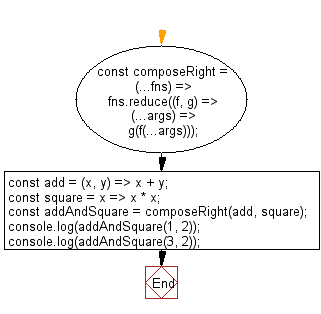# JavaScript: Perform left-to-right function composition

## JavaScript fundamental (ES6 Syntax): Exercise-67 with Solution

Write a JavaScript program to perform left-to-right function composition.

• Use Array.prototype.reduce() to perform left-to-right function composition.
• The first (leftmost) function can accept one or more arguments; the remaining functions must be unary.

Sample Solution:

JavaScript Code:

``````//#Source https://bit.ly/2neWfJ2
const composeRight = (...fns) => fns.reduce((f, g) => (...args) => g(f(...args)));
const add = (x, y) => x + y;
const square = x => x * x;

```
```

Sample Output:

```9
25
```

Pictorial Presentation:Flowchart:Live Demo:

See the Pen javascript-basic-exercise-67-1 by w3resource (@w3resource) on CodePen.

Improve this sample solution and post your code through Disqus

What is the difficulty level of this exercise?

Test your Programming skills with w3resource's quiz.

﻿

## JavaScript: Tips of the Day

Returns the difference (in days) between two dates

Example:

```const tips_DiffBetweenDates = (dateInitial, dateFinal) =>
(dateFinal - dateInitial) / (1000 * 3600 * 24);
console.log(tips_DiffBetweenDates(new Date('2020-05-13'), new Date('2020-06-05')));
```

Output:

```23
```